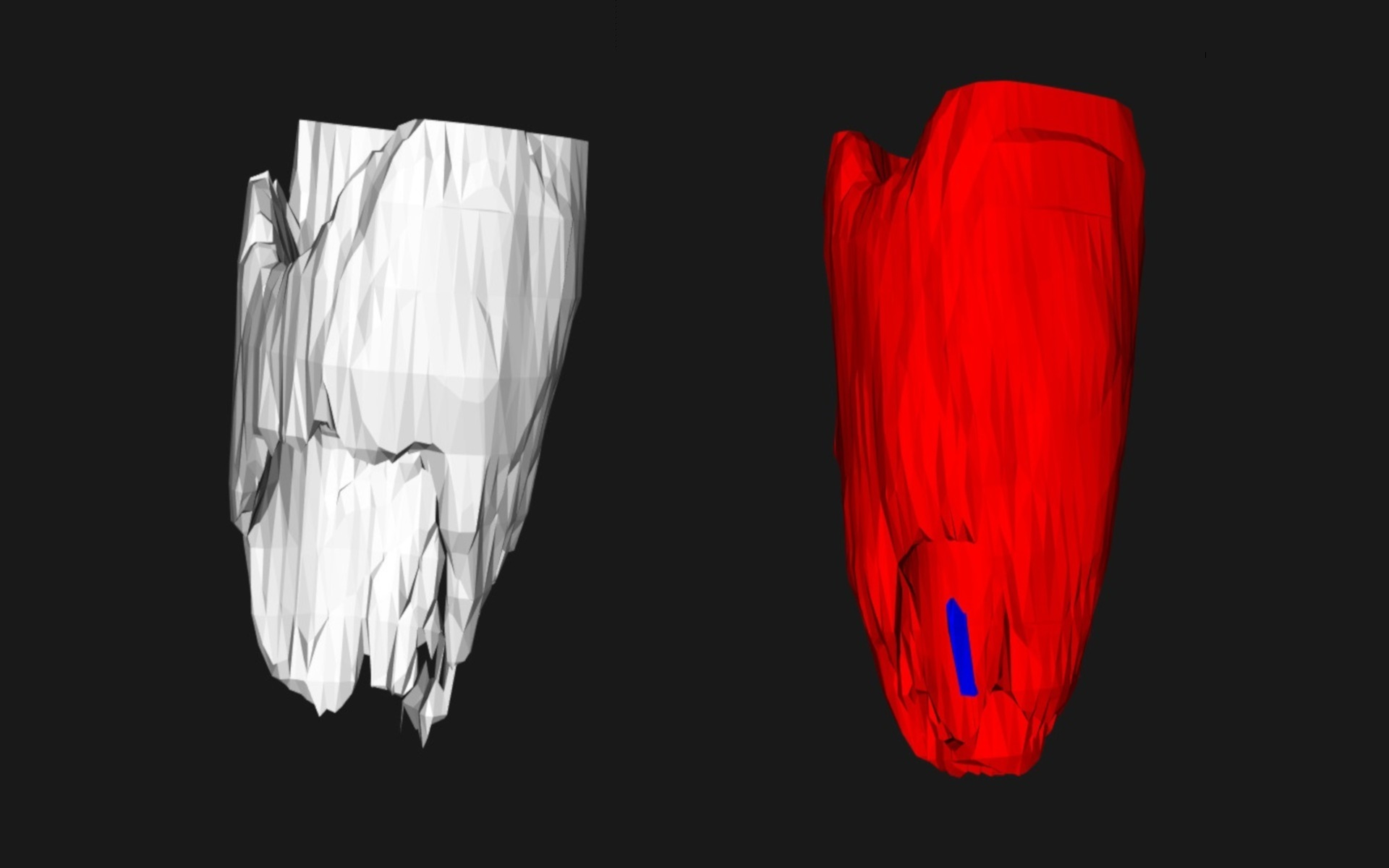Marching Tetrahedra Implementation
Date03/05/2017
Author(s)Andres Bejarano

Isosurface reconstruction using Marching Tetrahedra implemented in C++ and VTK.

After executing the program a console window will open asking for the isovalue for building the isosurface. Any number can be entered. It is recommended to work with values 100, 140, 150 and 200. Once the marching tetrahedra finishes building the isosurface the system will ask for a shell finding option. Two shell finding options are available: enter 1 for using vtkPolyDataConnectivityFilter (it finds the shells using a VTK filter and shows them with different colors), or enter 2 for a manual isosurface traversal. If option 2 is selected then the system will ask which shell should be displayed (shells will be numbered from 1 to n where n is the number of found shells). This options also displays in the console the number of triangles per shell.

A cube is subdivided into 6 tetrahedra, all of them having one diagonal in common. This approach avoids ambiguities while building the isosurface. Values between tetrahedron edges are calculated using inverse linear interpolation for the right parameter and then using linear interpolation for the 3D coordinates of the middle point. In some cases the interpolated points are reduced to a single point, such situation occurs when the value associated to a vertex is equal to the given isovalue, making the nominator in the inverse linear interpolation equal to zero. Such degenerate triangles are not considered for the isosurface reconstruction. For generating the shells an initial triangle is selected and it is asked for its neighbors (two triangles are neighbors if they have a common vertex).

The process is repeated for each neighboring triangle until all reachable triangles are visited. If there is any non-visited triangle left then the process is repeated using such triangle as the initial one. The number of obtained shells using this approach can be contrasted with the shells obtained by the vtkPolyDataConnectivityFilter object.